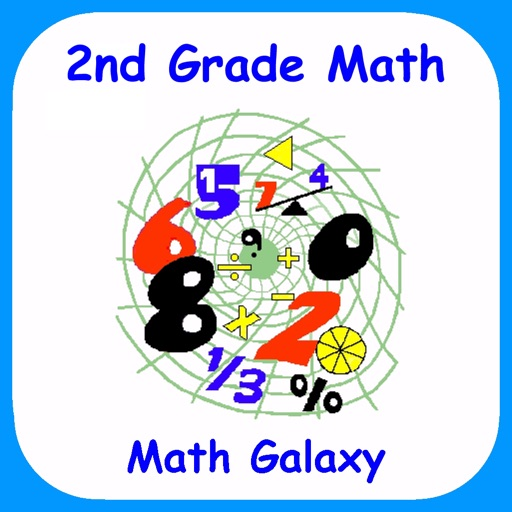"The French mathematician Jacques S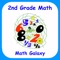2nd Grade Math - Math Galaxy

by Math Galaxy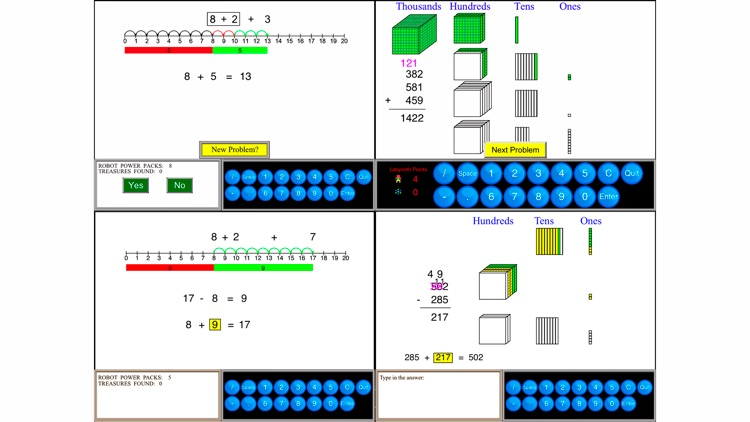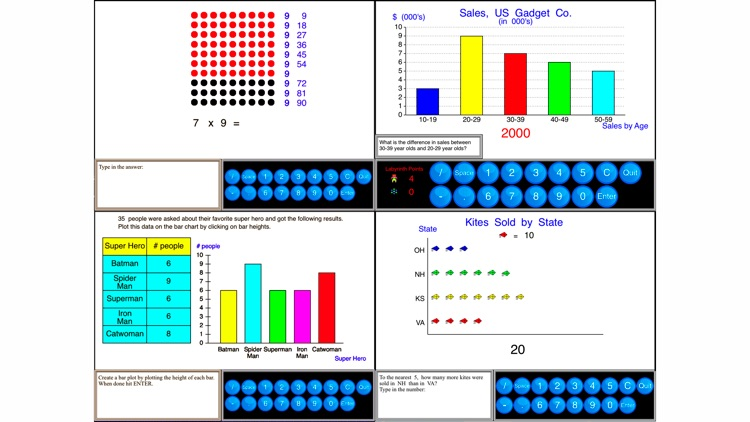"The French mathematician Jacques S. Hadamard found, in a study of 100 leading mathematicians [including Einstein], that the majority of them were primarily visual in their approach."App Details

Version
4.3
Rating
NA
Size
940Mb
Genre
Education Puzzle Board
Last updated
August 24, 2021
Release date
December 16, 2017

App Screenshots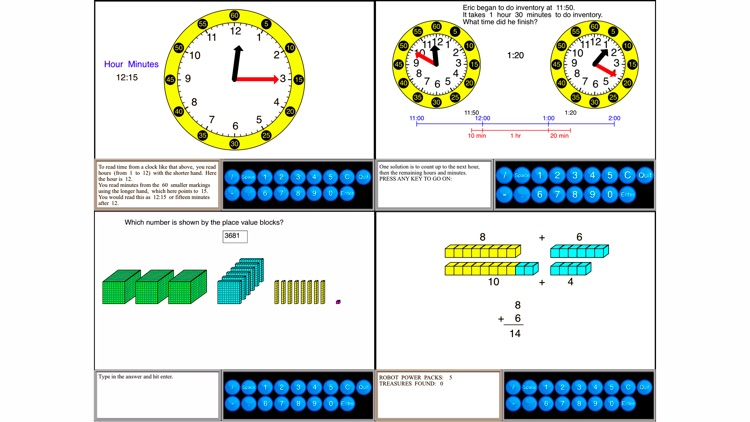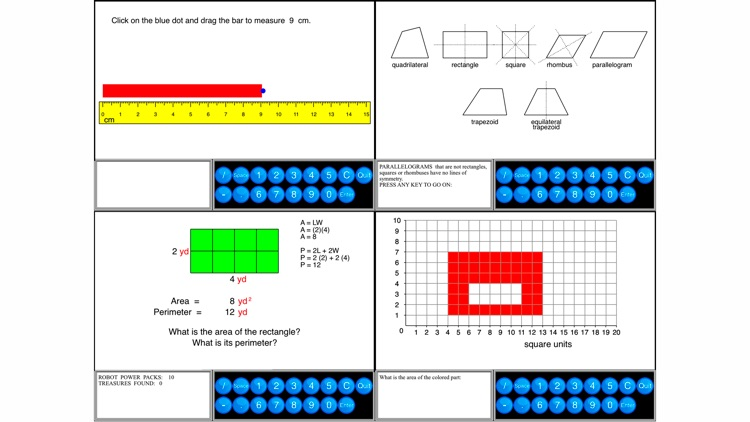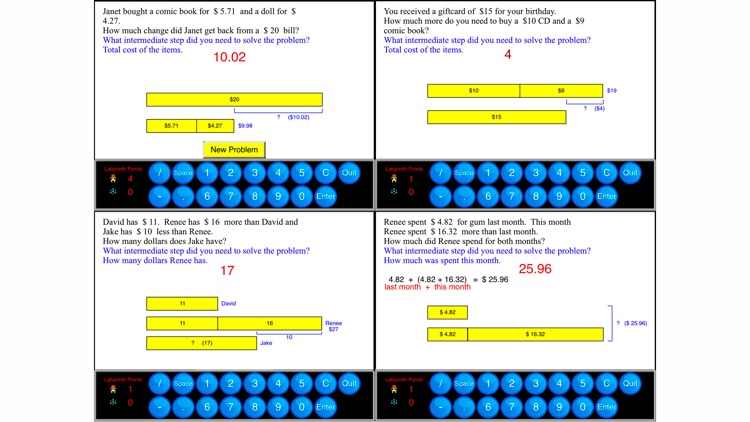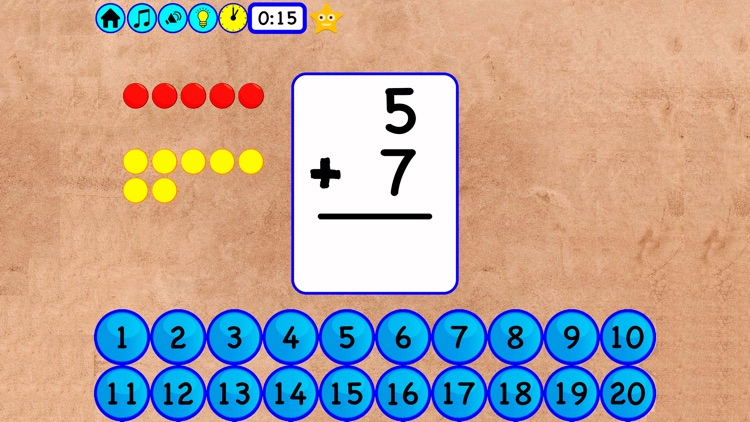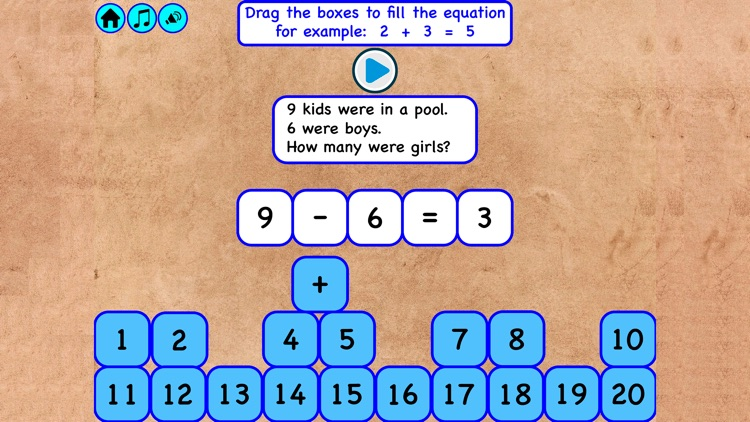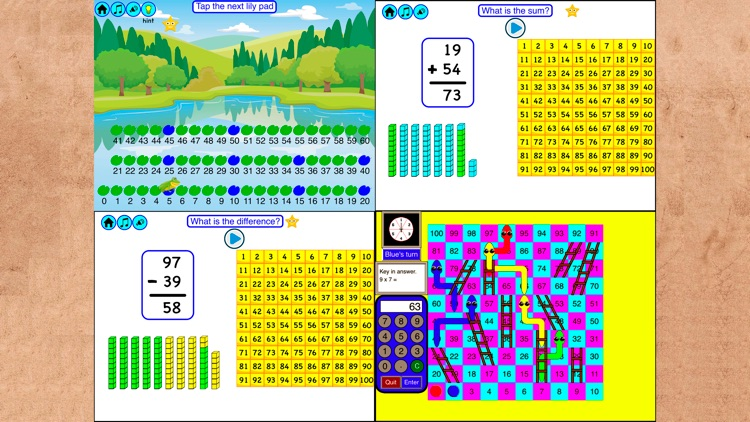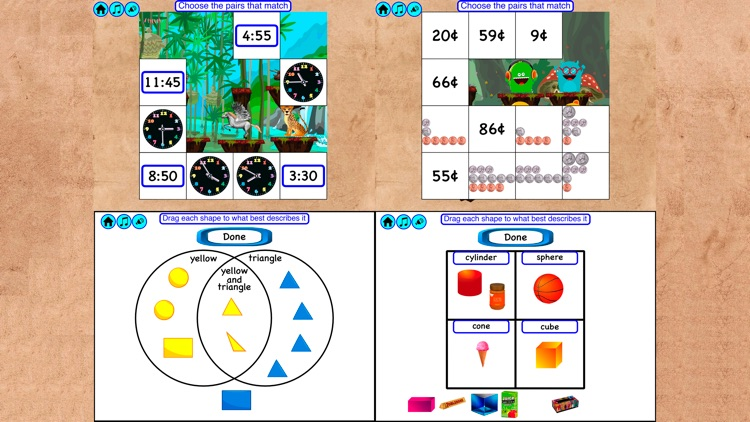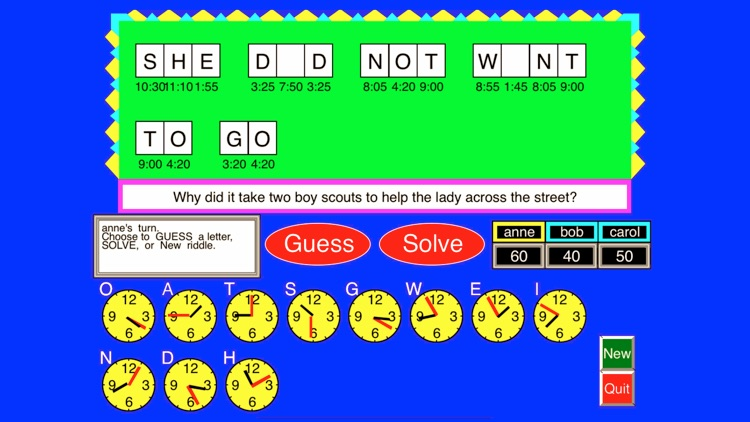App Store Description

"The French mathematician Jacques S. Hadamard found, in a study of 100 leading mathematicians [including Einstein], that the majority of them were primarily visual in their approach."
Scientific American, Sept., 1984, p. 56.

Math Galaxy’s Second Grade Math covers all Common Core math standards for second grade as listed below.
The concepts are presented in bite-size pieces with an unlimited supply of practice problems and numbers chosen randomly.
Illustrations are used throughout to reinforce the concepts.
There are review sections and you can be guided through problems step-by-step or just give the final answer.
Scores are recorded in Final-Answer mode.
By solving problems students earn robots to use in the Labyrinth maze game, plus there are several other games involving math practice.
Certificates with scores and summaries of scores can be emailed to be printed.
A stylus is recommended for the iPhone.

Now also supports Apple Schoolwork.

NUMBER AND OPERATIONS IN BASE 10 (2.NBT)

Understand place value. (2.NBT.A.1-4)

Place Value Blocks
Place Names
Expanded Notation
Comparing Numbers

Use place value understanding and properties of operations to add and subtract. (2.NBT.B.5-9)

Alternative Method
Subtraction on the Number Line
1-Digit Subtraction
2-Digit Subtraction
3-Digit Subtraction
Borrowing Across 0s
Missing Number
Alternative Method
Skip Counting (2.NBT.A.2)

OPERATIONS AND ALGEBRAIC THINKING (2.OA)

Represent and solve problems involving addition and subtraction

1-Step Word Problems (2.OA.A.1)
Multi-Step Word Problems (2.OA.A.1)

Add and subtract within 20. (2.OA.2)

Subtracting Whole Numbers

Work with equal groups of objects to gain foundations for multiplication

Multiplication Tables Up To 5 (2.OA.C.3-4)
Multiplication Tables - All (2.OA.C.3-4)

MEASUREMENT AND DATA (2.MD)

Measure and estimate lengths in standard units.

Measuring with Objects. (2.MD.A.1)
Measuring Inches & Centimeters (2.MD.A.1-3
Measuring in Standard Units
Measuring in Metric Units

Work with time and money.

Time - Clock Problems (2.MD.C.7)
Time - Word Problems (2.MD.C.7)
Math Games - Time Riddles (2.MDC..7)
Math Games - Money Riddles (2.MD.C.8)
Math Games - The MoneyTrail (2.MDC..8)

Represent and interpret data.

Measuring in Standard Units (2.MD.D.9)
Measuring in Metric Units (2.MD.D.9)
Pictographs (2.MDD..10)
Drawing Bar Graphs (2.MD.10)

GEOMETRY (2.G)

Reason with shapes and their attributes.

Shapes - Terminology (2.G.A.1)
Classify Shapes (2.G.A.1)
Area & Perimeter (2.G.A.2-3)
Missing Number
Area & Perimeter
Squares & Rectangles
Complex Areas
Fractions (2.G.A.3)

MATH GAMES

Labyrinth (Search mazes for treasure)